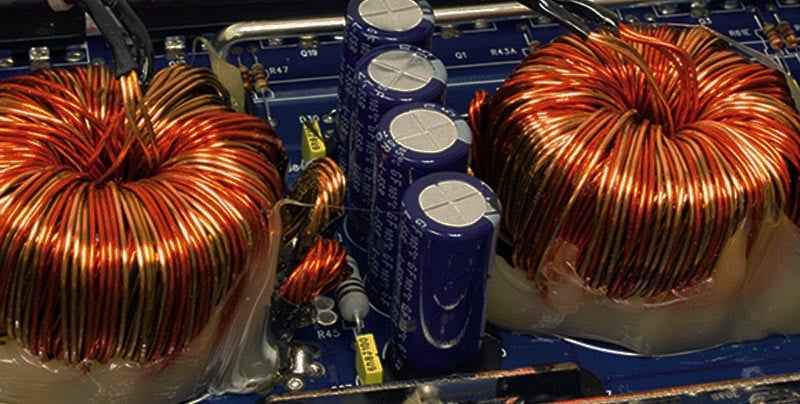# Fundamental Electric Circuits and Electronics Course for Beginner Engineers

Learn fundamentals of electric circuits and electronics, starting from the basics such as what an electric circuit is, electrical quantities like voltage, current & power, all the way to analyzing electric circuits.

Current Status
Not Enrolled
Price
\$28.45
Get Started

## Course Description

This course is designed to provide a complete overview of electric circuit analysis used in electrical engineering and electronics engineering. Electric circuit analysis is the most fundamental concept for electrical engineering, electronics engineering, and computer engineering.

It is for that reason that electric circuit analysis is usually the first course taught in electrical, electronics, and computer engineering programs at universities, as basically anything related to electrical, electronics, or computer engineering stems from electric circuit analysis.

In this course, you will learn everything about electric circuits and electronics, from the basics such as what an electric circuit is and the fundamentals of electrical quantities like voltage, current, and power, all the way to complex techniques for analyzing electric and electronic circuits.

The course is roughly divided into the following sections:

Fundamentals of Electric Circuits: in sections 2 and 3 of the course, we will discuss what an electric circuit is at the most basic level, followed by explanations of electrical quantities and sources of electricity. This is the foundation for electrical engineering and electronics engineering.

Basic DC Electric Circuit Analysis: in sections 4, 5, and 6 we will discuss the analysis of direct current (DC) circuits, starting from basic analysis techniques such as Kirchhoff’s voltage law and Kirchhoff’s current law (KVL and KCL), voltage division, current division, nodal analysis, and loop analysis. We will also be discussing how complex resistive circuits can be simplified into equivalent circuits for easier analysis of electric circuits and electronics.

Advanced DC Electric Circuit Analysis: in section 7, we will discuss advanced analysis techniques for electric circuits and electronics such as the superposition theorem, Thevenin’s theorem, and Norton’s theorem.

Energy-Storing Devices in Electric Circuits: in sections 8, and 9, we will discuss passive components in circuits that are able to store energy: capacitors and inductors. We will cover the fundamentals of capacitors and inductors, how they store energy, and how to simplify complex circuits containing combinations of capacitors and inductors into simpler circuits for easier analysis of electric circuits and electronics.

Transients in Electric Circuits: in section 10, we will discuss the analysis of first-order electric circuits during transients. This is where things start getting a bit more advanced, but we will solve several examples to illustrate how circuits behave during transients, as opposed to the stead-state circuits previously discussed.

In each section, several examples are solved to illustrate how to analyze practical circuits. By learning all the fundamentals about electric circuit analysis and electronics, you will be able to continue studying other topics in electrical engineering, electronics engineering, and computer engineering, such as analog electronics, digital electronics, circuit design, electric machines, power systems, and more.

## Course Overview

• Introduction To Electric Circuits and Electronics
• Sources in Electric Circuits and Electronics
• DC Resistive Electric Circuits
• Nodal Analysis Of DC Resistive Electric Circuits
• Loop Analysis of DC Resistive Electric Circuits
• Advanced DC Electric Circuits Analysis Techniques
• Capacitor and Their Uses in Electric Circuits and Electronics
• Inductors and Their Uses in Electric Circuits and Electronics
• First-Order Transient Electric Circuits
• AC Steady-State Electric Circuits Analysis

## Who Is This Course For

• Anybody with an interest in learning about electrical, electronics, or computer engineering. This is an introductory course, no prior engineering experience is required.

## Requirements

• Basic knowledge of algebra

• D.C. Circuits Concepts and Circuit Elements For Students (PDF)
• The Essentials Of DC Circuits - Methods Of Analysis, Laws and Theorems (PDF)

## Course Content

Lesson Content
0% Complete 0/3 Steps
Lesson Content
0% Complete 0/6 Steps
Lesson Content
0% Complete 0/4 Steps
Lesson Content
0% Complete 0/3 Steps
Lesson Content
0% Complete 0/3 Steps
Lesson Content
0% Complete 0/7 Steps
Lesson Content
0% Complete 0/7 Steps
Lesson Content
0% Complete 0/2 Steps

##### Ricardo Romero, PE

Hi, my name is Ricardo Romero and I am an Electrical Engineer with over ten years of experience in Power Systems. I obtained my Bachelor's and Master's degrees in Electrical Engineering from The University of North Carolina at Charlotte. I am also a licensed Professional Engineer in Power Systems in the states of Arizona, Louisiana, North Carolina, Pennsylvania, South Carolina, and Virginia in the United States. Although my professional experience is mainly in Power Systems, I am also passionate about other topics in Electrical Engineering including Analog Electronics, Control Systems, Digital Design, Digital Signal Processing, Electric Machinery, and Power Electronics.

13 Courses+27 enrolled
Not Enrolled

• 10 Lessons
• 66 Topics
• 8 Quizzes